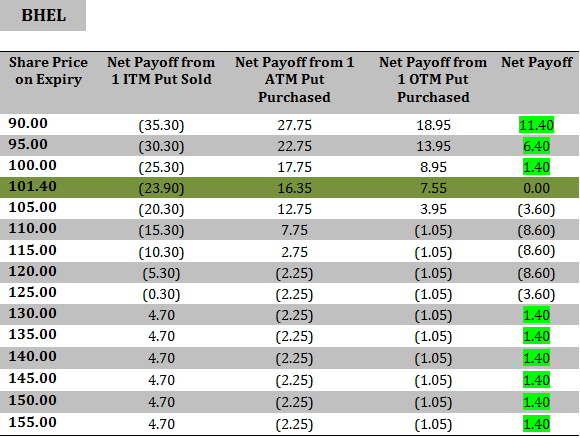When to use: Short Put Ladder Option Strategy is used when the investor is neutral to bullish on the stock and expects significant volatility.

How it works: In the short put ladder strategy you sell 1 in-the-money put option; buy 1 at-the-money put option and buy 1 out-of-the-money put option of the same underlying stock with the same expiry date. You believe that the market will be bullish until expiry.

For example: On 3rd September 2013, the share of BHEL was trading at Rs. 120.00, you decide to sell 1 in-the-money put option with a strike price of Rs. 130.00 at a premium of Rs. 4.70. At the same time you buy 1 at-the-money put option with a strike price of Rs. 120.00 at a premium of Rs. 2.25 and 1 out-of-the-money put option with a strike price of Rs. 110.00 at a premium of Rs. 1.05, all expiring on 26th September 2013.

For the short put option: If the price of the share stays above Rs. 130.00 (i.e. the strike price of the short put option) until expiry, you will retain the entire premium amount (i.e. Rs. 4.70). If however the price falls below Rs. 130.00, the buyer of the put option may exercise his option and make a profit based on how far below does the stock price fall. From the perspective of the seller of the put (you), you will start suffering a loss once the stock price falls below Rs. 125.30 (i.e. the strike price of the short put option – premium received).

For the long put options: If the price of BHEL falls below Rs. 120.00 and/or Rs. 110.00 (i.e. the strike prices for the 2 long put options), you can exercise your respective options, but the price of the stock must fall below Rs. 117.75 and/or Rs. 108.95 (i.e. strike price for the long put options – the amount of premium you paid for each option) for you to exercise your option and make a profit.

Risk/Reward: In the short put ladder strategy, your maximum risk will be limited, calculated as the difference in the strike prices (i.e. Rs 10.00) – net premium received. The maximum reward/profit which you stand to make from the short put ladder strategy will be limited if the underlying stock goes up and equals the net premium received. On the other hand, if the underlying stock moves downward, then the potential profit is unlimited due to the 2 long put positions. The breakeven point in this trade (i.e. Rs. 101.40) is calculated as: Sum of the strike prices of the two long puts – strike price of short put + net premium received.

The table below shows the net payoff of the Short Put Ladder Strategy at different spot prices on expiry:How to use the Short Put Ladder Option Excel calculator

Just enter your expected spot price on expiry, option strike price and the amount of premium, to estimate your net pay-off from the Short Put Ladder Option Strategy.

Note: The example and calculations are based assuming a single share though in reality options are based on lots of many shares. For example BHEL’s put option contract is for 2,000 shares. Accordingly the net premium received will be Rs. 2,800 for 3 lots (i.e. 1.40*2,000) in our example.

Also Note: Unlike the buyer of an option who only pays the premium to buy the option, the seller of an option must deposit a margin amount with the exchange. This is because he takes an unlimited risk as the stock price may rise to any level. In case the price rises sharply above the strike price, the exchange utilises the margin amount to make good the profit which the option buyer makes. The amount of margin is decided by the exchange and it typically ranges from 15 % to 60 % based on the volatility in the underlying stock and market conditions. In the above example, as a seller of call option, you will have to deposit a margin of Rs. 68,718.00 (i.e. Strike price * Lot size * 26.43%) for selling/writing a lot of BHEL’s put option. Note that the total value of your outstanding position in this case will be Rs. 2,60,000 (i.e. strike price * lot size).